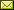## Super Exponentials in Linear Logic

 Esaïe Bauer (Univ Lyon, EnsL, UCBL, CNRS, LIP) Olivier Laurent (Univ Lyon, EnsL, UCBL, CNRS, LIP)

 Following the idea of Subexponential Linear Logic and Stratified Bounded Linear Logic, we propose a new parameterized version of Linear Logic which subsumes other systems like ELL, LLL or SLL, by including variants of the exponential rules. We call this system Superexponential Linear Logic (superLL). Assuming some appropriate constraints on the parameters of superLL, we give a generic proof of cut elimination. This implies that each variant of Linear Logic which appears as a valid instance of superLL also satisfies cut elimination.

In Ugo Dal Lago and Valeria de Paiva: Proceedings Second Joint International Workshop on Linearity & Trends in Linear Logic and Applications (Linearity&TLLA 2020), Online, 29-30 June 2020, Electronic Proceedings in Theoretical Computer Science 353, pp. 50–73.
Published: 30th December 2021.

 ArXived at: https://dx.doi.org/10.4204/EPTCS.353.3 bibtex PDF
References in reconstructed bibtex, XML and HTML format (approximated).
 Comments and questions to:eptcs@eptcs.org For website issues:webmaster@eptcs.org